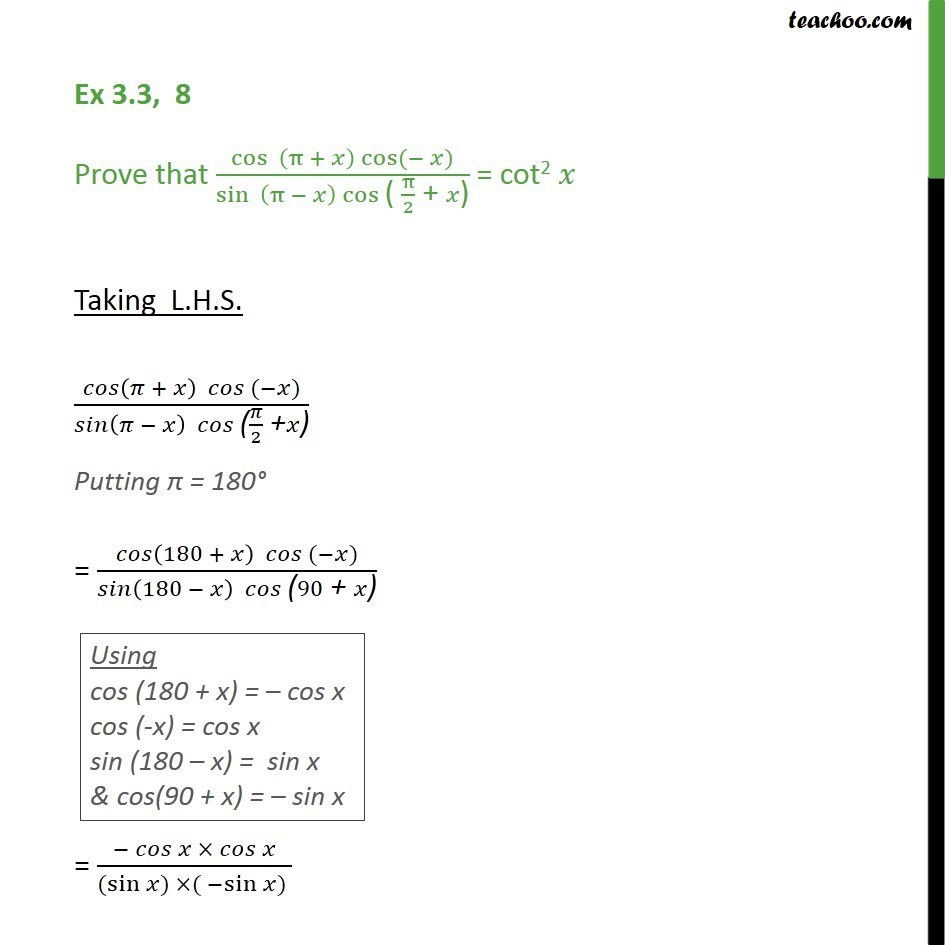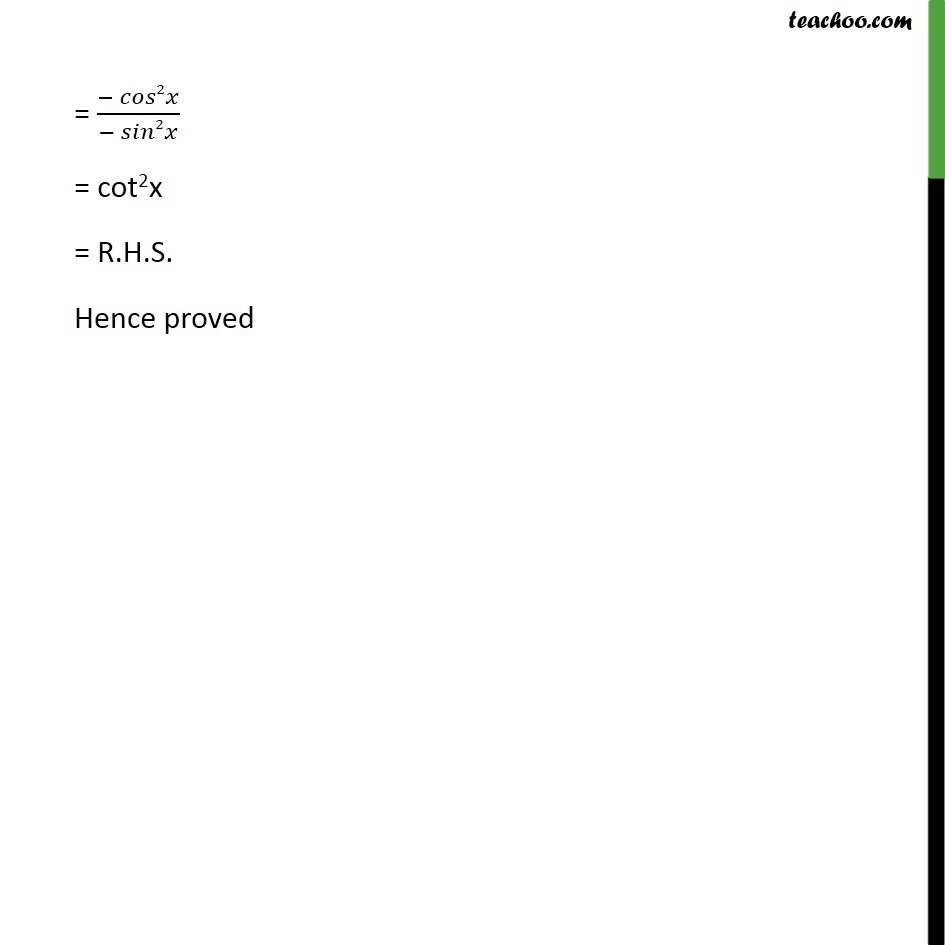1. Chapter 3 Class 11 Trigonometric Functions
2. Concept wise
3. Finding Value of trignometric functions, given angle

Transcript

Ex 3.3, 8 Prove that cos ( + ) cos ( ) /(sin ( ) cos "( " /2 " + " ")" ) = cot2 Taking L.H.S. ( + ) ( ) /( ( ) "(" /2 " +" ")" ) Putting = 180 = (180 + ) ( ) /( (180 ) "(" 90" + " ")" ) = ( )/((sin ) ( sin ) ) = ( 2 )/( 2 ) = cot2x = R.H.S. Hence proved

Finding Value of trignometric functions, given angle